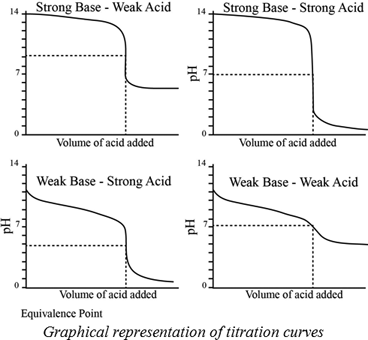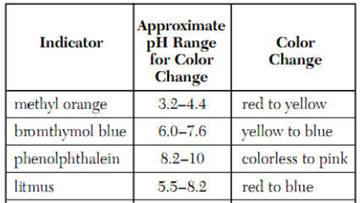Chapter 17, Problem 31PS

Chapter
Section
Textbook Problem

Without doing detailed calculations, sketch the curve for the titration of 30.0 mL of 0.10 M NaOH with 0.10 M HCl. Indicate the approximate pH at the beginning of the titration and at the equivalence point. What is the total solution volume at the equivalence point?

Interpretation Introduction

Interpretation:

The curve for titration of 30.0 mL of 0.10 M NaOH with 0.10 M HCl has to be sketched. The approximate pH at the beginning of the titration and at a equivalence point has to be indicated. And the total solution volume at the equivalence point has to be determined.

Concept introduction:

A titration is one of the most useful ways of determining accurately the quantity of an acid , a base or some other substances in a mixture.

The pH at the equivalence point of a strong acid –strong base titration is 7.

A weak acid titrated with a  strong base leads to a pH > 7 at the equivalence point.

A weak base titrated with a strong acid leads to pH < 7 at the equivalence point.Indicators:

A chemical substances which gives a visible change  in the titration.

Some important indicators are as follow.Explanation

1.0 M NaOH of pH is 14 and because of pH is logarithmic, a 1.0 M NaOH solution pH will be 13.

Here, we are using for titration acidic solution versus basic solution. Therefore, acidic solution will be acidic at high concentrations NaOH is strong bas and HCl is a strong acid. Therefore, the equivalence point at pH=7

Still sussing out bartleby?

Check out a sample textbook solution.

See a sample solution

The Solution to Your Study Problems

Bartleby provides explanations to thousands of textbook problems written by our experts, many with advanced degrees!

Get Started